Experiments with simulation of confinement of ions in a static electromagnetic field

12  Confinement of positive ions and electrons with a static electromagnetic field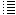Applying a reversed magnetic bottle

 A magnetic bottle has already been simulated  in:  Droom11.9.htmlThe idea was that it could help to confine the particles in the vertical direction. But could we do something with the magnetic field to help confine the particles more in the centre? Let´s reverse the magnetic bottle, making the diameter of the middle current-carrying ring smaller and the diameter of the outer current-carrying rings bigger and see what happens.. Experiment 12.1 Fig.1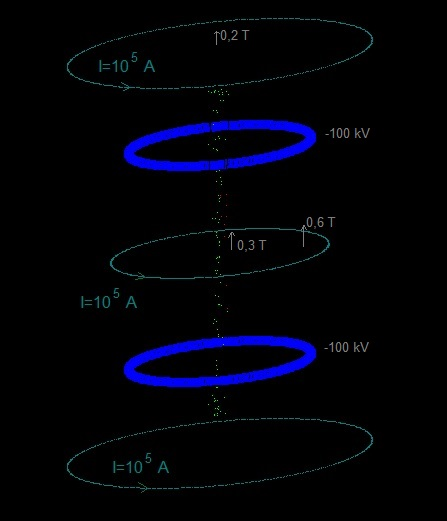Fig.2 . The image is augmented and the magnetic field direction and strength are visible.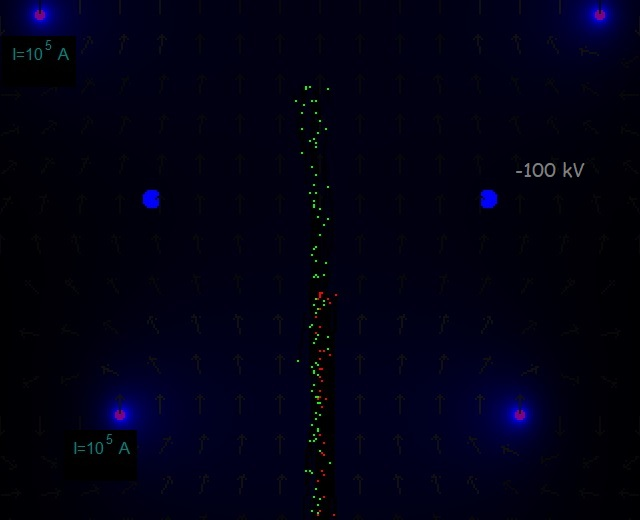Exp12.1.screenshot1The particles seem to be somewhat focused in the centre. If this is true, it could be an advantage, because it would increase the change that they colide and perhaps fuse in the centre. Fig.3 The upper and down current-carrying rings have been moved towards the centre.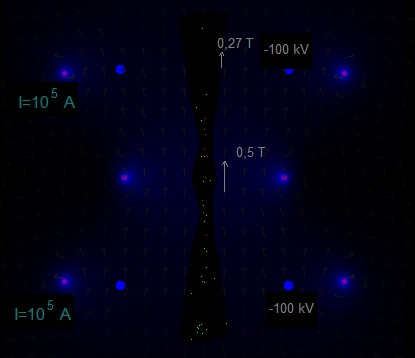Fig.4. A slightly different configuration.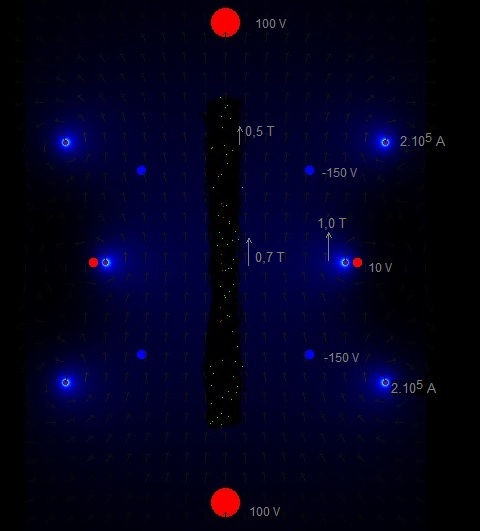Fig.5. Two charged rings with -150 kV and a bit stronger magnetic field..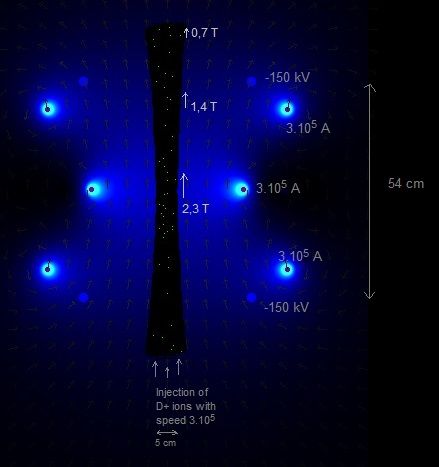Due to the configuration of the current-carying rings the magnetic field is curved. The charged particles (D+) ions tend to follow the magnetic field lines and are therefore somewhat squeezed towards the vertical central line (towards the centre). Fig.6.  The same as in fig.5, but the outer current-carrying rings have been moved a bit towards the centre, making the magnetic field more curved. (the lighter the color, the stronger the magnetic field)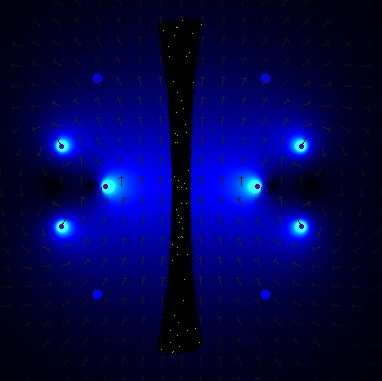The D+ ions reach a maximum vertical speed of about 1,5.106 m/s (see screenshot). Fig.7.  The same , but with the magnetic field even more curved. (the diameter of the middle ring has been decreased)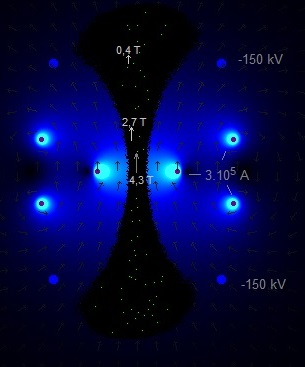The diameter of the middle current-carrying ring is quite small, and this should be difficult to realize. But it is just to see the effect of a curved magnetic field (a "reversed magnetic bottle") in the SEM fusor. It could  be that the particles are sucked in from above and from down under, but I am not sure of that. I did the same experiment again, but now only with 5 D+ ions. 4 D+ ions stayed in the upper and the under part, and only one D+ ion was moving up and down. But this one happened to be generated exactly in the vertical centre line.   Fig.8a. Another configuration.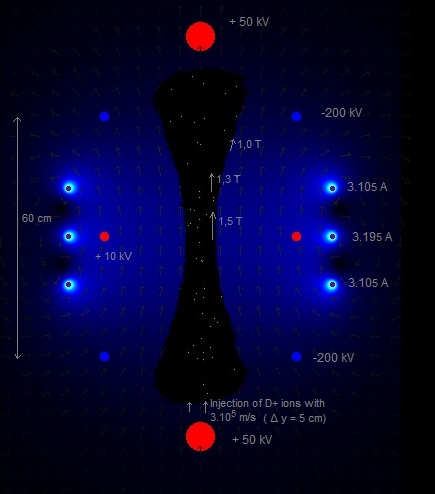Fig.8b. The same as fig. 8a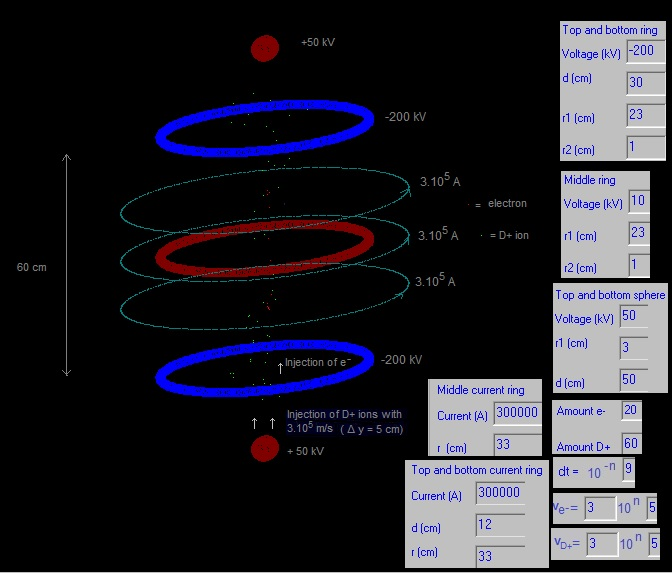It seems to be that some particles get trapped, more or less, in the area between the red spheres and the outer blue rings. This is probably because of the curved magnet field lines, by which some D+ ions will be bounched off. Hypothesis: The D+ ions will get all kind of velocities, due the collisions with other D+ ions. It could be that only those D+ ions that get, more or less, a vertical speed allongside the centreline will be accelerated  and move towards the centre of the vacuum chamber, where they will meet D+ ions from the other side with an opposite velocity. Perhaps the curved magnetic field between a red sphere and a blue ring will work as a kind of sieve, that only lets through D+ ions with the right velocity (more or less alongside the centre axis). This would be good for the SEM fusor! A lot of investigation and experiments can be done.... Fig. 9. Area between a red sphere and a blue ring with a curved magnetic field.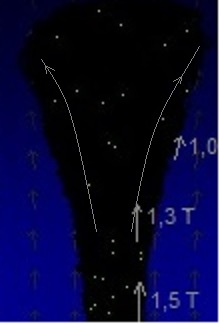---- How much is a current of 105 ampere? See also Droom11.4.htmlElectrical cable in Bricodepot.es:  2,5 mm2 -> 0,22 € / m According Engineeringtoolbox.com  the maximum current of such a cable is about 20 A. ->  105  = N. 20 -> N = 5000 turns Diameter of a turn = 60 cm -> circumference = 2π.0,3 = 1,89 m -> total length = 5000 . 1,89  m = 9450 m - > price = 2079 € . Fig. 3.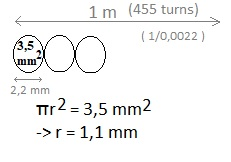If we take into account the insulation material around the cable (thickness of it is aproximated) and suppose the area of the cross section is then 3,5 mm2, then we can make over 1 meter length 1 layer with 455 turns. To get 5000 turns, we need about 11 layers. The thickness of all layers would be around: 2,4 cm. For copper the resistivity ρ = R . A/l  = 1,68 . 10-8  ohm meter  (R = electrical resistance, l = length, A = cross-sectional area) Diameter vacuum chamber = 50 cm, diameter coils = about 60 cm R =  ρ . l / A  = 1,68 . 10-8 . 5000 . 2. π . 0,30 / (  2,5 . 10-6  )     = 63 ohm Electrical power generated = I2 . R = 202 . 63 = 25,2 kW (about 25 electrical heaters of 1000 W) In the experiment of fig. 8 we have three rings with each carrying 3.105 ampere. So the total heat generated would be: 3*3*25,2 kW = 227 kW  (about 227 electrical heaters of 1000 W) Better use superconducting magnets...December 2019     by  Rinze Joustra        www.valgetal.com